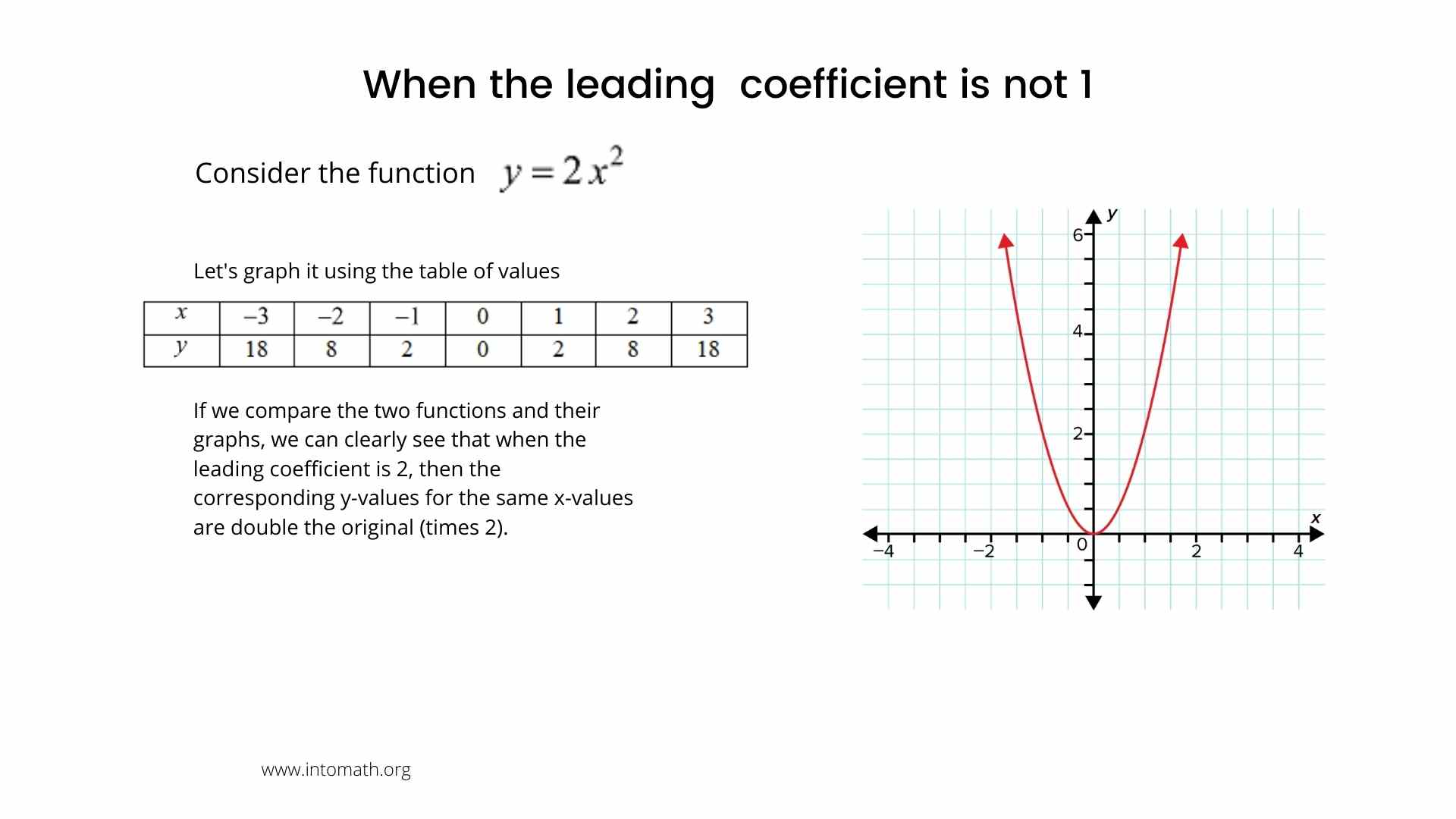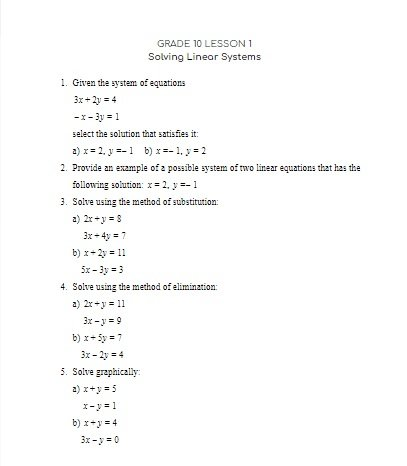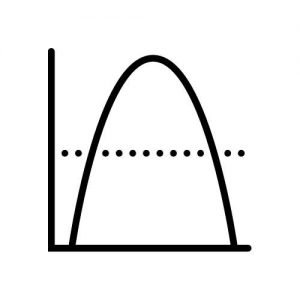CA$2.00 A quadratic function is a function of the form f(x) = ax2 + bx + c, where a cannot be 0. This is called a standard form equation. There are two other forms: vertex and factored. The graph of a quadratic function is called a parabola. Parabolas may open upward or downward. They have the “U” shape. The most basic parabola has an equation f(x) = x2. In order to graph this parabola, we can create the table of values, where x is the independent input and f(x) is the output of a squared input. The vertex of a parabola is its the highest or the lowest point. When a quadratic equation is given in the vertex form, it is easy to immediately determine the vertex by looking at the values of k and h. They are the coordinates of the vertex. f(x) = a(x – h)2 + k Vertex (h,k) Sometimes it is necessary to convert the standard form equation to a vertex form equation. In order to do that, we can use a process called “completing the square”. We can also complete the square geometrically. We can also use the method of completing a square to solve certain types of quadratic equations for zeros (x-intercepts) and determine where parabola intersects with the x-axis. Quadratic functions are very common in real life. Remember the Angry Birds game and movie? In order to design the game, the creators had to have a good understanding of a parabolic trajectory. A trajectory of a kicked ball or a diver diving off of the board are also described by parabolas. In basketball a quadratic functions are used to calculate the exact position of the player in order to get the ball in the net.Grade 10 Workbook (13 pages with answers) CA$4.00

A quadratic function is an important concept. Later in this grade we will also be learning how to solve quadratic equations by factoring and using the Quadratic Formula to find the x-intercepts.

Is the leading coefficient of the equation of this parabola a positive or a negative value?